Courses

SSC JE Civil Past Year Paper Technical - 2018 Notes | Study SSC JE Past Year Papers - Civil Engineering - Civil Engineering (CE)

Civil Engineering (CE): SSC JE Civil Past Year Paper Technical - 2018 Notes | Study SSC JE Past Year Papers - Civil Engineering - Civil Engineering (CE)

The document SSC JE Civil Past Year Paper Technical - 2018 Notes | Study SSC JE Past Year Papers - Civil Engineering - Civil Engineering (CE) is a part of the Civil Engineering (CE) Course SSC JE Past Year Papers - Civil Engineering.
All you need of Civil Engineering (CE) at this link: Civil Engineering (CE)

CIVIL & STRUCTURAL

Q.101. Which of the following represent the crushing strength (MPa) for the good quality stone that are used in the construction of buildings?
(a) Less than 20
(b) 20 to 60
(c) 60 to 80
(d) Greater than 100

Ans. (d)
Solution.
Crushing strength of a stone can be defined as the load per unit area at which the stone starts cracking. It should be greater than 100 N/mm2 to ensure sufficient strength for use in construction.

Q.102. Which of the following is examined to deter mine the age of timber?
(a) Annular ring
(b) Sapwood
(c) Pith
(d) Timber defects
Ans.
(a)
Solution.

The age of timber can be determined by counting the annual rings of wood growth.

Q.103. Which of the following is determined with the help of Le Chatelier’s device?
(a) Abrasion resistance
(b) Chemical resistance
(c) Soundness
(d) Strength
Ans.
(c)
Solution.

Soundness of cement indicates quality to expand on the setting whereas unsound cement expands too much on setting and develops cracks, distortion, and disintegration in the structure. Soundness of cement is tested by Le Chatelier’s device.

Q.104. The type of mortar which is used for the construction works carried out in water - logged area is _______.
(a) Cement mortar
(b) Loose mortar
(c) Mortar of very low consistency
(d) Mortar having high w/c ratio

Ans. (d)
Solution.
The mortar having high water cement ratio is used for the construction works carried act is water logged areas.

Q.105. For M 25 grade concrete, the split tensile strength in terms of percentage of its compressive strength is _______.
(a) 7 to 11%
(b) 18 to 28%
(c) 28 to 38%
(d) 38 to 48%

Ans. (a)
Solution.
M 25 grade concrete, this split tensile strength is terms of percentage of its compressive strength is 7 to 11%.

Q.106. Distempers are generally used to coat _______.
(a) Compound wall
(b) External concrete surfaces
(c) Interior surface which are not exposed to environment
(d) Wood works

Ans. (c)
Solution.
Distemper are generally in powdered form which is used to coat interior surface which are not exposed to environment.

Q.107. Which of the following is commonly used as retarder in cement?
(a) Calcium sulphate
(b) Gypsum
(c) Potassium carbide
(d) Sodium chloride
Ans.
(b)
Solution.

Retarders are admixture which prolong the chemical reaction between cement and water leading to increase is setting time. Following are the admixture used as a retarder are :- Gypsum, sugar, tartaric acid, lignin’s etc.

Q.108. In the process of hydration of OPC, to complete all chemical reaction, the water requirement (expressed as the percentage of cement) is _______.
(a) 5 to 8%
(b) 8 to 16%
(c) 20 to 25%
(d) 35 to 45%

Ans. (c)
Solution.
In the process of hydration of OPC, purely chemical requirements for the purpose of complete hydration of cement is about 20-25%

Q.109. The slump test is performed to check the _______.
(a) Presence of water in cement
(b) Ratio of concrete ingredients
(c) Temperature resistance
(d) Workability of concrete

Ans. (d)
Solution.
Workability of concrete can be measured by following methods are:-
(i) Slump test
(ii) Compacting factor test
(iii) Vee-Bee consistometer

Q.110. The reason behind the low expansion and shrinkage of the plywood is _______.
(а) Plies are placed at the right angles with each other
(b) They are glued under the high pressure
(c) They are held in the position with the help of adhesives
(d) They are prepared with the help of veneers

Ans. (a)
Solution.
In plywood, the piles are placed at the right angles with each other hence it has good strength both along and across the grains which leads to low shrinkage and low expansion.

Q.111. Which one of the following method is used for the approximate estimation?
(a) Both central line and short wall and long wall method
(b) Central line method
(c) Plinth area method
(d) Short wall and long wall method
Ans.
(c)
Solution.

The following are the methods used for preparation of approximate construction cost estimates : Plinth area method, cubical contexts methods and unit base method. It plinth are method, the cost of construction is determined by multiplying plinth area with plinth area rate. Plinth area rate are fixed with respect of quality and quantity of material and labour, type of foundation, height of buildings, roof, wood work, fix tunes, number of storeys etc.

Q.112. Accuracy in the measurement of the thickness of the slab or sectional dimension of column and beam (in centimetre) should be _______.
(a) 0.5
(b) 1
(c) 5
(d) 10
Ans.
(a)
Solution.

Accuracy required for the measurement of the thickness of the slab or sectional dimensions of column and beam should be 0.5 cm.

Q.113. Deduction at T-junction of the wall for total length of the central line is _______.
(а) Half of thickness of wall
(b) No deduction
(c) Thickness of wall
(d) Twice of the thickness of wall

Ans. (a)
Solution.
Taking out quantities for construction estimates by deduction of junction of the wall for total length of the central line for different junction are:
T-junctions:- Half (1/2) breadth wall measurement should be deducted for a T junction.
L-junctions:- No need to deduct from total centreline length.
Star junction:- Wall breadth measurement should be deducted to the total length of centreline.

Q.114. For estimation of painted area of semi corrugated asbestos cement sheets, percentage increase in area above plain area is _______.
(a) 0.1
(b) 0.14
(c) 0.2
(d) 0.25

Ans. (a)
Solution.
For estimation of painted area of semi corrugated asbestos cement sheet, percentage increase in area above plain area is 0.1%.

Q.115. Scrap value of a property may be _______.
(a) Both negative or positive
(b) Constant
(c) Negative
(d) Positive

Ans. (a)
Solution.
It is usually a positive number, but it could be negative if there is a cost of disposal, hence scrap value can be negative or positive.

Q.116. What is the unit of measuring cornice?
(a) Cubic metre
(b) Number
(c) Running metre
(d) Square metre

Ans. (c)
Solution.
Works consists of linear measurement involve length like cornice, fencing, hand rail, bands of specified width etc, are expressed in running metre (RM).

Q.117. Calculate the number of bricks in 20 cubic metres brick works.
(a) 500
(b) 1000
(c) 10000
(d) 100000

Ans. (c)
Solution.
Consider modular Brick,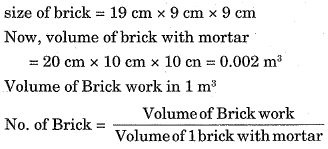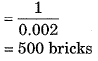No. of bricks for 20m3 brick work
= 20 x 500
= 10,000 Bricks

Q.118. Calculate the area (square metre) of the formwork required for a beam of 2 m span and cross section dimension of 400 mm x 200 mm.
(a) 0.8
(b) 0.16
(c) 1.2
(d) 2

Ans. (d)
Solution.
Beam we require: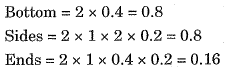Total requirements = 0.16 + 0.8 + 0.8 = 1.76 ≈ 2m2

Q.119. The cross section areas of three sections of an embankment at an interval of 40 m are 10 square metres, 15 square metres and 35 square metres. Calculate the quantity of earthwork for the embankment. Use prismoidal method.
(a) 1200
(6) 1400
(c) 1500
(d) 2400

Ans. (b)
Solution.
Prismoidal formula for volume (V)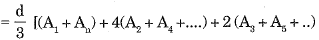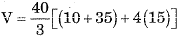V = 1400 m3.

Q.120. Calculate the annual depreciation (Rs.) of a machine having initial cost of Rs. 10,000. The scrap value is Rs. 1,000 and useful life of 30 years,
(a) 300
(b) 367
(c) 1333
(d) 333333

Ans. (a)
Solution.
Depreciation Rate for every year is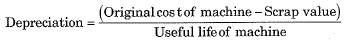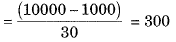Thus, the amount of depreciation of machine would be Rs 300.

Q.121. What is the difference between the sum of interior angles of plane triangle and spherical triangle for area of triangle 195 square kilometre on the Earth’s surface?
(a) One degree
(b) One minute
(c) One second

Ans. (c)
Solution.
There is difference of one second between the sum of interior angles of plane triangle and spherical triangle for area of triangle 195 km2 on the earth surface.

Q.122. Which one of the following is the largest scale?
(a)
1 : 500
(b)
1 : 1000
(c)
1 : 2500
(d)
1 : 50000
Ans. (a)

Q.123. The quadrantal bearing of the line lies in the third quadrant making angle in clockwise with the north is _______.
(a) N(θ - 180)°E
(6) N(θ - 180)°W
(c) S(θ - 180)°E
(d) S(θ - 180)°W

Ans. (d)
Solution.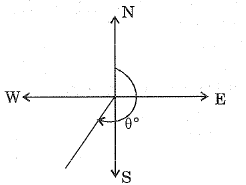Whole circle Bearing = θ
The quadrantal bearing of the line = S(θ - 180°)W

Q.124. Calculate the volume of the embankment (in cubic metre) using trapezoidal method, if the cross section areas of the three sections of an embankment at an interval of 30 m are 20 square metres, 40 square metres and 50 square metres.
(a) 1100
(b) 1150
(c) 2250
(d) 2350

Ans. (c)
Solution.
By trapezoidal method:-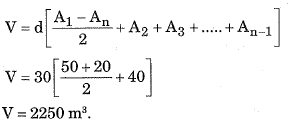Q.125. Which one is the CORRECT order of the tapes based on their accuracy?
(a) Linen tape > invar tape > metallic tape > steel tape
(b) Invar tape > steel tape > metallic tape > linen tape
(c) Metallic tape > steel tape > linen tape > invar tape
(d) Metallic tape > steel tape > Invar tape > linen tape

Ans. (b)
Solution.
Most accurate tape is Invar tape correct order:
Invar tape > Steel tape > Metallic tape > Linen tape.

Q.126. In the leveling between two points A and B on the opposite sides of a pond, the level is first set up near the point A and staff reading on A and B are 2.5 m and 2.0 m respectively. Then the level is moved and set near the point B, staff reading on points A and B are 1.2 m and 1.7 m respectively. Calculate the difference of heights between the two points A and B (in metre).
(a) 0
(b) 0.5
(c) 1
(d) 1.85

Ans. (a)
Solution.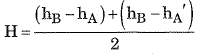When instrument at A:-
hA = Reading on staff at A = 2.5 m
hB = Reading on staff at B = 2.0 m
Whoa instrument at, B;
hA' = Reading on staff at A = 1.2 m
hB' = Reading on staff at B. = 1.7 m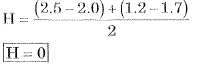Q.127. Calculate the combined correction for curvature and refraction (in m) for a distance of 2 km.
(а) 0.045
(b) 0.135
(c) 0.269
(d) 3.14

Ans. (c)
Solution.
Combined connection Cc = -0.06728 d2
d = 2km = Distance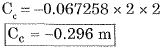Q.128. In transit theodolite, the line of the sight can be reversed by revolving the telescope through _______.
(a) 90° in horizontal plane
(b) 90° in vertical plane
(c) 180° in horizontal plane
(d) 180° in vertical plane

Ans. (d)
Solution.
In transit theodolites, the line of right can be reversed by revolving the telescope through 180° in vertical plane.

Q.129. Which one is the CORRECT sequence for the temporary adjustment of the theodolite?
(a) Centering, elimination of parallax, leveling, and setting
(b) Centering, setting, elimination of parallax and leveling
(c) Setting, centering, leveling and elimination of parallax
(d) Setting, leveling, elimination of parallax and centering

Ans. (c)
Solution.
Sequence of temporary adjustment of theodolite is
(i) Setting of instrument
(ii) Centering of instrument
(iii) Levelling the instrument by the help of bubble tube
(iv) Elimination of parallax

Q.130. Which of the following is used for determining the location of station occupied by the plane table?
(b) Intersection method
(d) Two point problem

Ans. (d)
Solution.
Reaction is process of determining the location of the station (on the map) occupied by the plane table.
(i) Resection by two point problem
(ii) Resection by three point problem.

Q.131. Which of the following is responsible for the formation of residual soil?
(a) Glaciers
(b) Water
(c) Wind
(d) None of these

Ans. (d)
Solution.
Residual soils are formed when soils or rocks weather at the same location due to chemical, water and other environmental elements without being transported.

Q.132. The coefficient of gradation and the coefficient of uniformity of a given soil sample is 1.0 and 4.0 respectively. The ratio of effective size to the diameter through which 30% of the total mass is passed is _______.
(a) 1.25
(b) 1.5
(c) 1.75
(d) 2

Ans. (d)
Solution.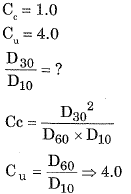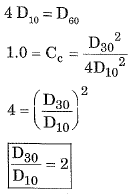Q.133. Which of the following shows the CORRECT order of increasing surface areas of the given soil?
(a) Clay < silt < sand < colloids
(b) Gravel < silt < colloids < clay
(c) Sand < silt < clay < colloids
(d) Silt < gravel < colloids < clay

Ans. (c)
Solution.
Increasing order of surface areas of given soil: Sand < Silt < Clay < Colloids

Q.134. What is the assumption made about back of wall, in the Rankine's theory of earth pressure?
(a) Plane and rough
(b) Plane and smooth
(c) Vertical and rough
(d) Vertical and smooth

Ans. (d)
Solution.
Assumptions of Rankine theory:
(i) The soil mass in homogenous, isotropic, semifinite, elastic, dry and cohesionless.
(ii) The ground surfaces in planar which may be horizontal or inclined.
(iii) The face of wall in contact with backfill is vertical and smooth.
(iv) The soil is in the state of plastic equilibrium in positive and active earth pressure condition.
(v) The rupture surface is planar.

Q.135. Which of the following is CORRECT about the viscosity of gas?
(a) Inversely proportional to the temperature
(b) Increases with an increase in the temperature
(c) Independent of pressure
(d) Independent of temperature

Ans. (b)
Solution.
Viscosity of liquids decreases with temperature where as viscosity of gases increases with increase in temperature.

Q.136. Pressure of 200 kPa is equivalent to the head of z metre of liquid having relative density 1.59. The value of z (m) is _______.
(a) 11.6
(b) 11.82
(c) 12.82
(d) 13.14

Ans. (c)
Solution.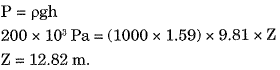Q.137. Which one of the following statement is CORRECT about the center of buoyancy?
(a) It is the point where buoyant force act.
(b) It coincides with the centroid of volume of water displaced
(c) It is the point where buoyant force act. and It coincides with the centroid of volume of water displaced
(d) It acts outside the body.

Ans. (c)
Solution.
Center of buoyancy is the point where the buoyant force act and it coincide with the centroid of volume of water displaced.

Q.138. A longitudinal rectangular surface is hanged into the water such that its top and bottom points are at depth of 1.5 m and 6.0 m respectively. The depth of center of pressure (m) from the top surface is _______.
(a) 3.8
(b) 4.2
(c) 4.6
(d) 4.8

Ans. (b)
Solution.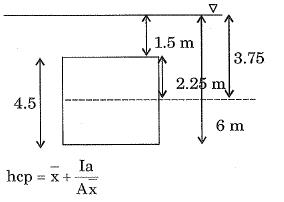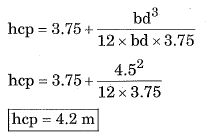Q.139. The velocity potential which follow the equation of continuity is _______ , _______.
(a) x2y
(b) x2 - y2
(c) cos x
(d) x2 + y
2
Ans. (b)
Solution.

Equation of continuity: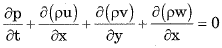According to given conditions: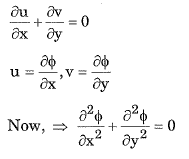Case 1:
If ϕ = x2y
⇒ 2 ≠ y + 0 ≠ 0
Case 2:
If ϕ = (x2 - y2)
⇒ +2 - 2 = 0

Q.140. At what distance from the boundary layer, the value of the wall shear is three times of the turbulent shear?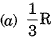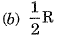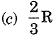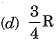Ans. (c)
Solution.
Wall shear stress is 3 times of the turbulent shear, when the distance from the boundary layer is (2/3)R.

Q.141. Which of the following statement is CORRECT about the stream lines and equipotential lines?
(a) Both can be drawn graphically for viscous flow around any boundary.
(b) Meshes formed by them are always squares.
(c) They always meet orthogonally.
(d) They can be calculated for all boundary conditions.

Ans. (c)
Solution.
Streamliner and equipotential lines always acts orothogonally.

Q.142. The Rankine half oval body MM is subjected to the two-dimensional flow having velocity V. The typical stream line is shown in the following diagram. The point A in diagram shows _______.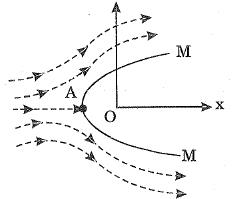(a) Point at which velocity is maximum
(b) Separation point
(c) Stagnation point
(d) Stall point

Ans. (c)
Solution.
Stagnation point is a point is a flow field where the local velocity of the fluid is zero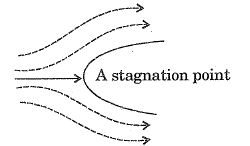Separation point is defined as the point between the forward and backward flow where the shear stress is zero.
Stall is a condition in aerodynamic and aviation such that if the angle of attack increase beyond a certain point then lift begins to decrease.

Q.143. Which of the following is CORRECT ratio for Froude number?
(a) Compressive force to inertia force.
(b) Inertia force to gravity force.
(c) Inertia force to tension force.
(d) Viscous force to inertia force.

Ans. (b)
Solution.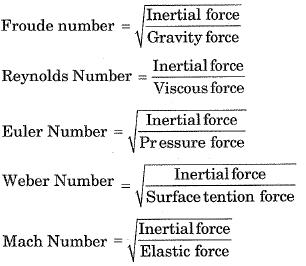Q.144. For the most economical triangular channel section, the angle of sloping sides from the vertical is.
(a) 30°
(b) 45°
(c) 60°
(d) 75°

Ans. (b)
Solution.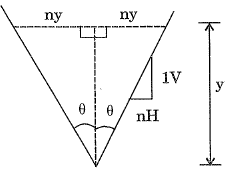Let us consider a triangular section with side slope 1 V : nH. Depth of flow is y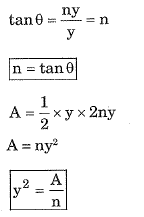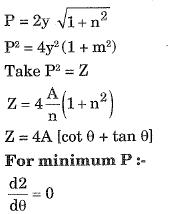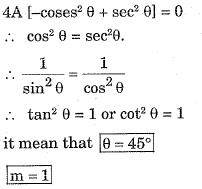Q.145. Method of applying water directly to the root zone of the plant is called _______.
(a) Check flooding
(b) Drip method
(c) Furrow method
(d) Sprinkler irrigation

Ans. (b)
Solution.
Check flooding:
(i) In check flooding the crop area is divided into some plots which are relatively leveled by checks or bunds
(ii) Water from field channels is allowed to enter to each plots or check basin and the plots are flooded to the required depth.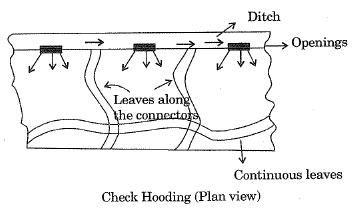Check flooding (plan view)
Drip Method: Drip irrigation is a type of micro-irrigation system that has the potential to save water and nutrients by allowing water to drip slowly be the roots of plants, either from above the soil surface or buried below the surface. The goal is to place water directly into the root zone and minimize preparation.
(i) Furrow irrigation: It is a type of irrigation in which trenches or ‘furrow’ are dug between crop row in a field farmer flow water down the furrows and it seeps vertically and horizontally to refill the soil reservoir.
(ii) Sprinkler irrigation: Sprinkler irrigation is the method of applying water in a controlled manner in way similar to rainfall.

Q.146. A field of 500 hectares is to be irrigated for a particular crop having 100 days base period. The total depth of water required by the crop is 100 cm. Calculate the duty of the water (in hectares per cubic metre).
(a) 8.64
(b) 57.87
(d) 86.4
(d) 864

Ans. (d)
Solution.
Base period B = 100 days
Depth of water A = 100 cm = 1 m.
Duty of the water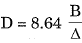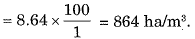Q.147. The traffic volume of a roadway is defined as the multiplication of _______.
(b) Speed and distance way
(c) Traffic density and speed

Ans. (c)
Solution.
Traffic density = K
Speed of which = V
Traffic volume = q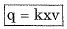Q.148. Calculate the equivalent radius (cm) of the resisting section of 20 cm slab, if the ratio of radius of wheel load distribution to the thickness of the slab is 2.
(a) 20
(b) 35.6
(c) 40
(d) 40.9

Ans. (c)
Solution.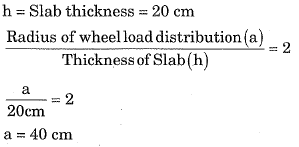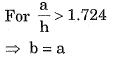Hence b = equivalent radius of resisting section = 40 cm

Q.149. On peak hourly demand, what is the maximum daily consumption for the city which have average daily consumption of 100,000 m3?
(a) 140000
(b) 170000
(c) 200000
(d) 270000

Ans. (d)
Solution.
Maximum Hourly
Demand of maximum day
= 2.7 x (Annual Average hourly demand)
= 2.7 x 100,000
= 270000 m3

Q.150. For which of the following, distribution mains is designed?
(a) Average daily demand
(b) Annual peak demand
(c) Monthly peak demand
(d) Maximum hourly demand on maximum day

Ans. (d)
Solution.
Service reservoir and distribution mains are designed for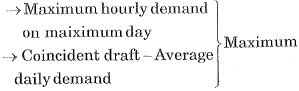Q.151. Which of the following statements is true?
A. Most of the loads applied to a building are environmental load.
(a) Only A
(b) Only B
(c) Both A and B
(d) Neither A nor B

Ans. (b)
Solution.

Q.152. How does an increase in the pitch of the roof affects the amount of load that can be placed on it?
(a) It increases
(b) It decreases
(c) Remains constant
(d) Depends upon case

Ans. (b)
Solution.
The heating load is found to increase with the roof pitch, so the amount by load in decreased that can be placed on it.

Q.153. What will be the rain load (in psf) if ds is 2 inches and dh is 1 inches?
(a) 5.2
(b) 10.4
(c) 15.6
(d) 20.8

Ans. (c)
Solution.
As per ASCE 7,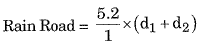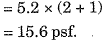Q.154. Concrete is:
(а) Good in compression, good in tension
(b) Good in compression, weak in tension
(c) Weak in compression, weak in tension
(d) Weak in compression, good in tension

Ans. (b)
Solution.
Concrete is a brittle material. It is good in compression but weak in tension.

Q.155. If in planar system, X parts/members are there with Y number of forces, then condition for statically determinacy is:-
(a) Y < 3X
(6) Y > 3X
(c) Y = 3X
(d) None of these

Ans. (c)
Solution.
X = parts / members
Y = No. of forces
In planar system, the statically determinacy is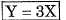Q.156. If a system has more equations of equilibrium than number of forces, then the system is:
(a) Improperly constrained
(b) Partially constrained
(c) Stable
(d) None of these

Ans. (b)
Solution.
Partially constrained, if it has two or fewer reaction compound (There is not enough reaction to prevent motion under all possible loading condition). Improperly constrained, if has three or more reaction.

Q.157. Which of the following material is not used in making trusses?
(a) Wooden struts
(b) Metal bars
(c) Channel
(d) Concrete

Ans. (d)
Solution.
Concrete cannot be used for making trusses because trusses undergo reversal of stresses and concrete is very weak in tension.

Q.158. In a truss it is assumed that the members are joined by
(a) Rough pins
(b) Smooth pins
(c) Either rough or smooth pins
(d) None of these

Ans. (b)
Solution.
Assumption for truss analysis:
(i) The member cannot develop moments at the ends forces.
(ii) The connections to other member are perfectly pinned/hinged through frictionless (smooth) pips.
(iii) Each member is of uniform cross sectional area.
(iv) The entire structure is in one plane if it is a plane truss.
(v) Load act at the joints only. Self weight of the truss is neglected. It the member are connected at the ends with gusset plates and welded, no fixity is assumed.

Q.159. What is the major difference between truss and beam?
(а) Beam can’t transmit load in vertical direction while truss can
(b) Truss can’t transmit load in vertical direction while beam can
(c) Beam can’t transmit load in axial direction while truss can
(d) Truss can’t transmit load in axial direction while beam can

Ans. (b)
Solution.
Truss member are always subjected to axial loads while beam is subjected to axial force, shear force and bending moments.

Q.160. Given that J is number of joints. B and R are no. of members and number of reactions.
If B = 4, R = 3 and J = 4, then the truss is:
(a) Statically determinate
(b) Statically indeterminate and stable
(c) Stable
(d) Unstable

Ans. (d)
Solution.
Ds = m + r - zj
m = B = No. of member = 4
r = R = No. of reaction = 3
j = J = No. of joints = 4
Ds = 4 + 3 - (2 x 4)
Ds = -1 < 0 (unstable)

Q.161. Which IS code gives details regarding water to be used in concrete?
(a) IS 456
(b) IS 383
(c) IS 565
(d) IS 3012

Ans. (a)
Solution.
IS 456 - 2000 gives details regarding mix design and water used in concrete.

Q.162. Which of the below is an example of plasticizer?
(a) Hydroxylated carboxylic acid
(b) Fluoro-silicate
(c) Gypsum
(d) Surkhi

Ans. (a)
Solution.
Hydroxylate carboxylic acid, lignosulfonates are common plasticizers.

Q.163. How many methods of batching of concrete, are there?
(a) 2
(6) 3
(c) 5
(d) 6

Ans. (a)
Solution.
There are only method of batching of concrete
(i) Volume Batching: In volume batching materials are measured on the basis of volume.
(ii) Weight Batching: The batching is done measured on the basis of weight.

Q.164. Concrete is generally placed on a:
(а) Form work
(b) Stand
(c) Mould
(d) Platform

Ans. (a)
Solution.
(i) Form work is temporary or permanent molds into which concrete or similar materials are poured.
(ii) Moulds are used to make something into a particular shape or form by pressing it or by putting it into a mould.
(iii) For working at Height the working platform is used.

Q.165. The effective width of a column strip of a flat slab is taken as
(a) One-fourth the width of the panel
(b) Half the width of the panel
(c) Half the diameter of the column
(d) The diameter of the column

Ans. (b)
Solution.
As per IS 456: 2000
The effective width of a column strip of a flat slab is taken as half the width of panel.

Q.166. Permanent dimension changes due to loading of concrete is termed as:
(a) Strain
(b) Extent
(c) Creep
(d) Ambit

Ans. (c)
Solution.
Permanent and some temporary dimension changes due to constant load at constant temperature for long duration of time is called as creep.

Q.167. In design of R.C.C. structures, the tensile strength of concrete is taken as:
(a) 5N/mm2
(6) 2N/mm2
(c) 0.3N/mm2
(d) None of these

Ans. (d)
Solution.
Concrete does not take up tensile loads, it is taken as zero (We basically design cracked section). But IS 456 - 2000 recommends the tensile strength to be calculated using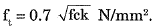It is bending (flexural) tensile strength.

Q.168. Flexure strength of concrete is determined as:
(a) Modulus of rigidity
(b) Modulus of rupture
(c) Modulus of plasticity
(d) Modulus of elasticity

Ans. (b)
Solution.
Flexural strength or modulus of rupture of concrete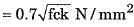Q.169. Properties of concrete can broadly be divided into:
(a) 1
(6) 4
(c) 2
(d) 3

Ans. (c)
Solution.
Based on the state of concrete, its properties vary. Concrete in the fresh state has different properties than concrete in a hardened state.

Q.170. Which IS code gives specifications about cement plaster?
(a) IS 1500
(b) IS 1221
(c) IS 1400
(d) IS 1661

Ans. (d)
Solution.
IS 1661 → Code of proactive for the application of cement and cement-time plastering on building
IS 1542 : 1992 → Specification for sound for plaster
IS 1500 - (2005) → Method for Brinell Hardness Test.

Q.171. In a lime - cement plaster, ratio 1:1:6 corresponds to:
(а) Lime : cement : sand
(b) Cement : Lime : sand
(c) Lime : sand : gravel
(d) Cement : sand : gravel

Ans. (b)
Solution.
Lime - Cement plaster, ratio is given as - Cement : Lime : Sand

Q.172. On which of the following does the correct proportion of ingredients of concrete depend upon?
(a) Bulking of sand
(b) Water content
(c) Absorption and workability
(d) All options are correct
Ans. (d)
Solution.
Factor affecting the Design mix of concrete:
(i) Compressive strength of concrete
(ii) Workability of concrete
(iii) Durability of concrete
(iv) Water-Cement Ratio
(v) Maximum Nominal size of aggregrate
(vii) Quality control at site
(viii) Bulking of sand.

Q.173. If X, Y and Z are fineness moduli of coarse fine and combined aggregates, the percentage (P) of fine aggregates to combined aggregates is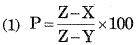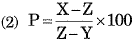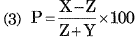(a)  only
(b)  only
(c)  only
(d)  only
Ans. (b)
Solution.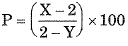X = Fineness modulus of coarse aggregrate
Y = FM of fine aggregrate
Z = FM of com bined.

Q.174. Which of the following statements is false?
(а) Workability of the concrete mix decreases with an increase in the moisture content
(b) Concrete for which preliminary tests are conducted, is called controlled concrete
(c) Bulking of sand depends upon the fineness of grains
(d) All options are correct
Ans.
(d)
Solution.

(i) Concrete for which preliminary test are conducted, is called controlled concrete
(ii) Bulking of sand depend upon the fineness of grains
(iv) Work ability of concrete is inversely proportional to water cement ratio because if the amount of w/c ratio increases, workability decreases.

Q.175. Which of the following statements is false?
(a) Space between the exterior walls of a warehouse and bag piles should be 30 cm
(b) Cement bags should preferably be piled on wooden planks
(c) Cement bags should be placed such that bags of one layer does not touch the bags of the adjacent layer
(d) None of these

Ans. (d)
Solution.
Following points are kept in mind for cement warehouse:
(i) Space between the exterior walls of a warehouse and bag piles should be 30 cm
(ii) Cement bag should be placed closer together in the piles.
(iii) Width and height of the piles should not exceed 3 m and 2.70 m respectively

Q.176. Which of the following statements is false?
(a) With passage of time, the strength of cement increases
(b) With passage of time, the strength of cement decreases
(c) After a period of 24 months, the strength of cement reduces to 50%
(d) The concrete made with storage deteriorated cement gains strength with time

Ans. (a)
Solution.
Strength of concrete increases with time but for cement decreases with time.

Q.177. For a concrete mix 1:3:6 and water cement ratio 0.6 both by weight, what is the quantity of water required per bag?
(a)
10 kg
(b)
12 kg
(c)
14 kg
(d)
None of these
Ans. (c)

Q.178. Transport of concrete by pumps, is done for a distance of
(a) 100 m
(b) 200 m
(c) 300 m
(d) 400 m

Ans. (d)
Solution.
Transporting of concrete by pump is done for horizontal distance 400 m and vertical distance of 90 m. Pumped with a rate of 8 to 70 m3/hour.

Q.179. The compression in PSC is done by _______ : _______ of high-strength tendons.
(a) Compression
(b) Tensioning
(c) Shearing
(d) Bending

Ans. (b)
Solution.
By tensioning the tendons in pre stressed concrete it undergoes compression

Q.180. In which beam tension capacity of steel is greater than combined compression capacity of steel and concrete?
(a) Over-reinforced
(b) Under-reinforced
(c) Singly reinforced
(d) Doubly reinforced

Ans. (b)
Solution.
In under-reinforced section
(i) ta = fst
(ii) Ca < σcbc (characteristic strength of concrete)

Q.181. If W is total load per unit area on a panel, D is the diameter of the column head, L is the span in two directions, then the sum of the maximum positive bending moment and average of the negative bending moment for the design of the span of a square flat slab, should not be less than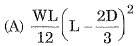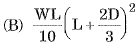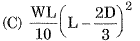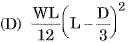(a) A only
(b) B only
(c) C only
(d) D only
Ans. (c)

Q.182. For a circular slab carrying a uniformly distributed load, the ratio of the maximum negative to maximum positive radial moment is
(a) 1
(b) 2
(c) 3
(d) 4
Ans. (b)
Solution.
Maximum negative radial moment  = 3WR2/16
Maximum positive radial moment = 6WR2/16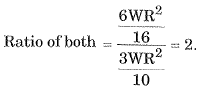Q.183. If permissible compressive stress in concrete is 15 kg /cm2, tensile stress in steel is 1400 kg /cm2 and modular ratio is 18, the depth of the beam is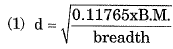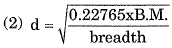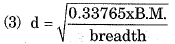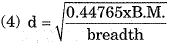(a)  only
(b)  only
(c)  only
(d)  only
Ans. (a)
Solution.
Compressive strength of concrete σcbc = 50 kg/cm2.
Tensile stress in steel = 1400 kg/cm2
Modulus Ratio m = 18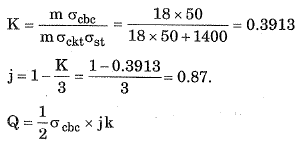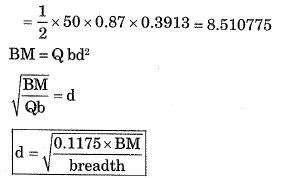Q.184. The breadth of a ribbed slab containing two bars must be between
(a) 6 cm to 7.5 cm
(b) 8 cm to 10 cm
(c) 10 cm to 12 cm
(d) None of these

Ans. (b)
Solution.
(i) Clear spacing between rips shall not be greater than 4.5 cm.
(ii) Width of the rib shall not be less than 7.5 cm
(iii) Overall depth of the slab shall not exceed four times the breadth of the rib
(iv) The thickness of the topping of a ribbed slab varies between 5 cm to 8 cm.
(v) The breadth of a ribbed slab containing two bars must be between 8 cm to 10 cm.

(vi) The maximum diameter bar used in ribbed slab is 22 mm.

Q.185. A foundation rests on which of the following?
(a) Base of the foundation
(c) Foundation soil
(d) Both Sub grade and foundation soil
Ans.
(d)
Solution.

Foundation rests on both subgrade soil and foundation soil.

Q.186. Which of the following statements is true?
(a) To ensure uniform pressure distribution, the thickness of the foundation is kept uniform throughout
(b) To ensure uniform pressure distribution, the thickness of the foundation is increased gradually towards the edge
(c) To ensure uniform pressure distribution, the thickness of the foundation is decreased gradually towards the edge
(d) To ensure uniform pressure distribution, the thickness of the foundation is kept zero at the edge

Ans. (c)
Solution.
The maximum load acts at centre line of footing or centre of foundation. Intensity of load in reduced towards the outer edge of footing, footing is designed according to load distribution, thickness of fasting is maximum at centre and reduced towards edge of footing. But pressure distribution will be uniform throughout width of footing.

Q.187. The weight of a foundation is assumed as which of the following?
(a) 5% of wall weight
(6) 7% of wall weight
(e) 10 % of wall weight
(d) 12 % of wall weight

Ans. (c)
Solution.
Weight of foundation generally taken as 10% of wall weight or column load.

Q.188. If the width of the foundation for two equal columns is restricted, the shape of the footing generally adopted is
(a) Square
(b) Rectangular
(c) Trapezoidal
(d) Triangular

Ans. (b)
Solution.
Rectangular shaped footing is generally adopted if the width of the foundation for two equal columns is restricted.

Q.189. Maximum shear stress theory for the failure of a material at the elastic limit is known as
(a) Guest’s or Trecas’ theory
(b) St.Venant’s theory
(c) Rankine’s theory
(d) Haig’s theory

Ans. (a)
Solution.
(i) Maximum principle stress theory-Rankine theory.
(ii) Maximum principle strain theory-St. Venant’s theory.
(iii) Maximum shear stress theory-Guest and Tresca’s theory.
(iv) Maximum strain energy theory-Haigh’s theory
(v) Maximum shear strain theory- Distortion theory Mises Henky theory.

Q.190. A simply supported beam carries a varying load from zero at one end and w at the other end. If the length of the beam is a, the maximum bending moment will be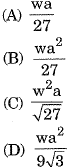Ans. (d)
Solution.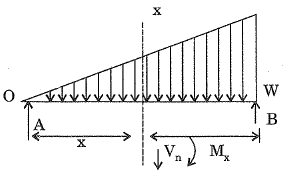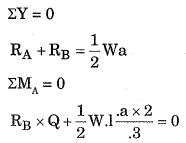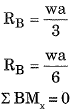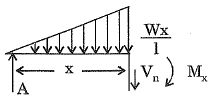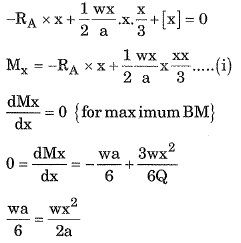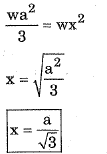Put value of x in equation (i) we go →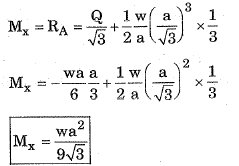Q.191. When not specified, the volume of steel in R.C.C. work is taken as:-
(a) 1% to 1.6% of R.C.C. volume
(b) 2% to 4% of R.C.C. volume
(c) 4% to 6% of R.C.C. volume
(d) 0.6 % to 1% of R.C.C. volume
Ans. (d)
Solution.
If not specified, the volume of steel in RCC work is taken as 0.6% to 1% OF RCC volume.

Q.192. The ratio of maximum shear stress to average shear stress of a circular beam is:
A. 2/3
B. 3/2
C. 3/4
D. 4/3
(a) A only
(b) B only
(c) C only
(d) D only
Ans. (d)

Solution.
Maximum shear stres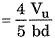= 4/3  average shear stress

Q.193. The property of a material by which it can be beaten or rolled into plates, is called
(a) Ductility
(b) Plasticity
(c) Elasticity
(d) None of these
Ans. (a)
Solution.
Property of a material by which it can be beaten or rolled into plates is called malleability.

Q.194. What is the limit to Poisson's ratio?
(a) 0.1
(b) 0.2
(c) 0.3
(d) None of these
Ans. (d)
Solution.
Limiting value of Poisson’s Ratio are - 1 < μ < 0.5.

Q.195. Among the following, which is least elastic?
(a) Iron
(b) Copper
(c) Both Copper and Silver
(d) Rubber

Ans. (d)
Solution.
Rubber is least elastic materials.
Young modulus of iron = 162 - 170 GPa
or
Modulus of elasticity
Youngs modulus of silver = 85 GPa
Young modulus of copper = 128 GPa
Young modulus of rubber < Silver < Copper < Iron

Q.196. Two bars of different materials are of the same size and are subjected to same tensile forces. If the bars have unit elongations in the ratio of 4 : 7, then the ratio of modulius of elasticity of the two materials is
A. 4:7

B. 4:10
C. 16:49
(a) A only
(b) B only
(c) C only
(d) None of these

Ans. (d)
Solution.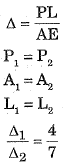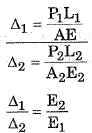Q.197. If a composite bar of steel and copper is heated, then the copper bar will be under:
(a) Tension
(b) Shear
(c) Torsion
(d) None of these

Ans. (d)
Solution.
In composite bar the final length of bath bars must remain same. As we know, thermal coefficient of copper is more than steel, but the final length to be compressive force is induced in copper bar.

Q.198. Pick up the incorrect statement from the following : The torsional resistance of a shaft is directly proportional to
(a) Modulus of rigidity
(b) Angle of twist
(c) Reciprocal of the length of the shaft
(d) Moment of inertia of the shaft section.

Ans. (d)
Solution.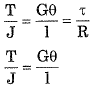Q.199. Net sectional area of a tension member, is equal to its cross section area _______ .
(a) Plus the area of the rivet holes
(b) Divided by the area of rivet holes
(c) Multiplied by the area of the rivet holes
(d) Minus the area of the rivet holes

Ans. (d)
Solution.
Net sectional area = Area of cross section - Area of Rivet holes

Q.200. When a tension member consists of two channel sections, the allowance for rivet hole is made for two holes from
(a) Each web
(b) Each flange
(c) Each web or one hole from each flange whichever is more
(d) Each web or one hole from each flange whichever is less

Ans.
Solution.
If a tension member consists of two channel section, the allowance for rivet holes is made for two holes from each web or one hole from flange whichever is less.

The document SSC JE Civil Past Year Paper Technical - 2018 Notes | Study SSC JE Past Year Papers - Civil Engineering - Civil Engineering (CE) is a part of the Civil Engineering (CE) Course SSC JE Past Year Papers - Civil Engineering.
All you need of Civil Engineering (CE) at this link: Civil Engineering (CE)Use Code STAYHOME200 and get INR 200 additional OFF Use Coupon Code

Top Courses for Civil Engineering (CE)SSC JE Past Year Papers - Civil Engineering

10 docs

Top Courses for Civil Engineering (CE)Track your progress, build streaks, highlight & save important lessons and more!

,

,

,

,

,

,

,

,

,

,

,

,

,

,

,

,

,

,

,

,

,

;